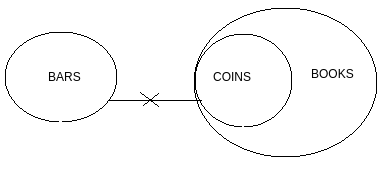# Directions : Given two statements, verify the conclusions and mark the answer as given below. Statements: I.          No bars are coins                                  II.          All coins are books Conclusions: I.          All coins are books                   II.          Some books are not bars   Option 1)   if only conclusion I follow.   Option 2)    If only conclusion II follows.   Option 3)    If either conclusion I or II follows.   Option 4)   If neither of the two conclusions follows.     Option 5)   If both conclusions follow